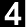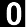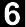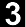Pedosphere 29(2): 224--234, 2019
ISSN 1002-0160/CN 32-1315/P
©2019 Soil Science Society of China
Published by Elsevier B.V. and Science Press
 Characterizing Spatial Variability of Soil Textural Fractions and Fractal Parameters Derived from Particle Size Distributions Meysam MOHAMMADI1, Mahmoud SHABANPOUR1, Mohammad Hossein MOHAMMADI2, Nasser DAVATGAR3 1Department of Soil Science, Faculty of Agriculture, University of Guilan, Rasht 41889-58643 (Iran)2Department of Soil Science, Faculty of Agricultural Engineering and Technology, University of Tehran, Karaj 31587-77871(Iran)3Department of Soil Science, Research Institute of Soil and Water, Karaj, Meshkin Dasht 31779-93545 (Iran) ABSTRACT Soil particle size distribution (PSD) is a fundamental physical property affecting other soil properties. Characterizing spatial variability of soil texture is very important in environmental research. The objectives of this work were:1) to partition PSD of 75 soil samples, collected from a flat field in the University of Guilan, Iran, into two scaling domains using a piecewise fractal model to evaluate the relationships between fractal dimensions of scaling domains and soil clay, silt, and sand fractions and 2) to assess the potential of fractal parameters as an index used in a geostatistical approach reflecting the spatial variability of soil texture. Features of PSD of soil samples were studied using fractal geometry, and geostatistical techniques were used to characterize the spatial variability of fractal and soil textural parameters. There were two scaling domains for the PSD of soil samples. The fractal dimensions of these two scaling domains (D1 and D2) were then used to characterize different ranges of soil particle sizes and their relationships to the soil textural parameters. There was a positive correlation between D1 and clay content (R2=0.924), a negative correlation between D1 and silt content (R2=0.801), and a negative correlation between D2 and sand content (R2=0.913). The geometric mean diameter of soil particles had a negative correlation with D1 (R2=0.569) and D2 (R2=0.682). Semivariograms of fractal dimensions and soil textural parameters were calculated and the maps of spatial variation of D1 and D2 and soil PSD parameters were provided using ordinary kriging. The results showed that there were also spatial correlations between D1 and D2 and particle size fractions. According to the semivariogram models and validation parameters, the fractal parameters had powerful spatial structure and could better describe the spatial variability of soil texture. Key Words:  fractal dimension,geometric mean diameter,geostatistical approach,physical property,semivariogram model,statistical correlation Citation: Mohammadi M, Shabanpour M, Mohammadi M H, Davatgar N. 2019. Characterizing spatial variability of soil textural fractions and fractal parameters derived from particle size distributions. Pedosphere. 29(2):224-234. View Full TextCopyright © 2019 Editorial Committee of PEDOSPHERE. All rights reserved.
Address: P. O. Box 821, 71 East Beijing Road, Nanjing 210008, China    E-mail: pedosphere@issas.ac.cn
Technical support: Beijing E-Tiller Co.,Ltd.